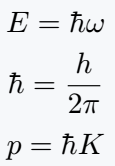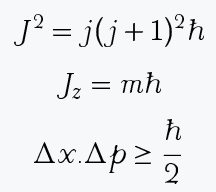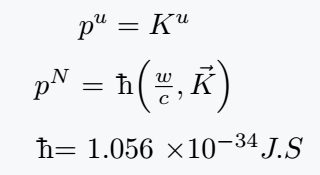# How do you type the h-bar(ℏ) symbol in LaTeX?

You can print the h-bar or Reduced Plank constant by default \hbar command in the latex document.

Symbol H-bar
Type of symbol Mathematics
Package (requirement) No
Argument No
Latex command \hbar
Example \hbar → ℏ
\documentclass{article}
\begin{document}
$E = \hbar \omega$
$\hbar = \frac{h}{2\pi}$
$p = \hbar K$
\end{document}

Output :And different packages have the same command, which returns the same output. Examples are amsmath, fdsymbol, and boisik.

There is another type of h-bar symbol in latex that has structural light differences. For this, take help of boisik package.

\documentclass{article}
\usepackage{boisik}
\begin{document}
$J^2 = j(j+1)^2 \hslash$
$J_z = m \hslash$
$\Delta x . \Delta p \geq \frac{\hslash}{2}$
\end{document}

Output :## Use another package for h-bar symbol in LaTeX like tipa, wsuipa, phonetic, etc.

The following program uses four different packages and different commands to define the same h-bar symbol.

Command (package) Symbol
\textcrh (tipa)
\crossh (wsuipa)
\plank (phonetic)
\textcrh (t4phonet)

\documentclass{article}
\usepackage{tipa, phonetic, wsuipa}
\begin{document}
$p^u = \textcrh K^u$\\[6pt]
$p^N$ = \planck $\left(\frac{w}{c}, \vec{K} \right)$\\[6pt]
\crossh = 1.056 $\times 10^{-34} J.S$
\end{document}

Output :When you came from the Last Paragraph of this tutorial, then I hope you’ve got your answer like the mind that you wanted.#### Md Jidan Mondal

LaTeX expert with over 10 years of experience in document preparation and typesetting. Specializes in creating professional documents, reports, and presentations using LaTeX.